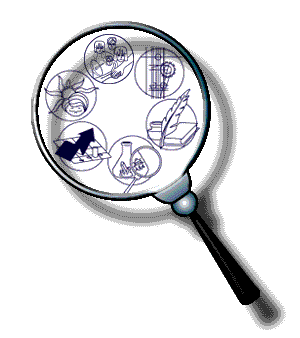# Dinar

Home | IndexWe love those sites:

```2 definitions found

From The Collaborative International Dictionary of English v.0.48 [gcide]:

Dinar \Di"nar\ (d[imac]"n[~e]r or d[-e]*n[aum]r"), n. [Ar.
d[imac]n[=a]r, from Gr. dhna`rion, fr. L. denarius. See
{Denier}.]
1. A petty money of accounts of Persia; 100 dinars consituted
a rial.
[1913 Webster]

2. An ancient gold coin of the East, issued by various
Islamic countries.
[1913 Webster]

3. the unit of currency of Yugoslavia. One dinar equals 100
paras. Abbreviated Din.
[PJC]

From WordNet (r) 2.0 [wn]:

dinar
n 1: 100 dinars equal 1 rial [syn: {Iranian dinar}]
2: the basic unit of money in Yugoslavia [syn: {Yugoslavian
dinar}]
3: the basic unit of money in Tunisia [syn: {Tunisian dinar}]
4: the basic unit of money in Libya [syn: {Libyan dinar}]
5: the basic unit of money in Kuwait; equal 1,000 fils [syn: {Kuwaiti
dinar}]
6: the basic unit of money in Jordan; equal to 1,000 fils [syn:
{Jordanian dinar}]
7: the basic unit of money in Iraq; equal to 1,000 fils [syn: {Iraqi
dinar}]
8: the basic unit of money in Bahrain; equal to 1,000 fils
[syn: {Bahrain dinar}]
9: the basic unit of money in Algeria [syn: {Algerian dinar}]
```